Science, Maths & Technology

### Become an OU student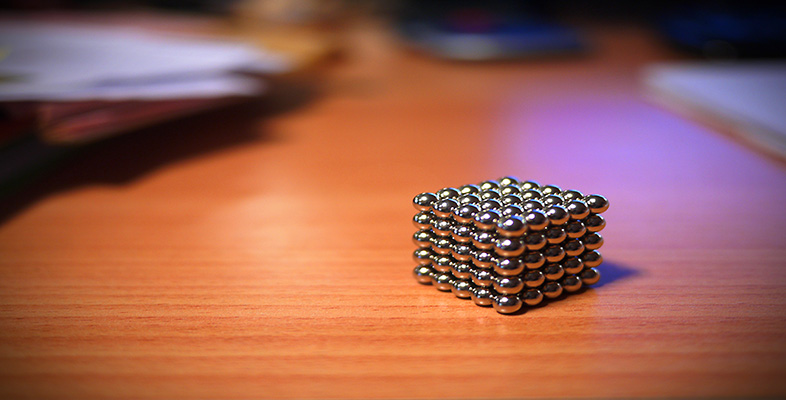Squares, roots and powers

Start this free course now. Just create an account and sign in. Enrol and complete the course for a free statement of participation or digital badge if available.

# 1.4.1 Try some yourself

## Activity 8

If a litre is one cubic decimetre, how many litres are there in a cubic metre?

Since a litre is one cubic decimeter, the question is asking how may cubic decimetres are in a cubic metre.

1 metre = 10 decimetres

so 1 m3 = 103 cubic decimetres.

Hence there are 1000 litres in a cubic metre.

## Activity 9

Find the following without using your calculator, as an estimate for the calculator work in the next question.

• (a) (1)3

• (b) 33

• (c) 1003

• (d) 0.13

• (a) (1)3 = 1 × 1 × 1 = 1 × 1 = 1

• (b) 33 = 3 × 3 × 3 = 9 × 3 = 27

• (c) 1003 = 100 × 100 × 100 = 10 000 × 100 = 1000 000 (a million)

• (d)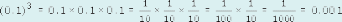## Activity 10

Use your calculator to find the following.

• (a) (1.2)3

• (b) 3.33

• (c) 1013

• (d) 0.1213

• (a) Estimate: (1)3 = 1.

Calculate: (1.2)3 = 1.728.

• (b) Estimate: 33 = 27.

Calculate: 3.33 = 35.937.

• (c) Estimate: 1003 = 1000 000.

Calculate: 1013 = 1030 301.

• (d) Estimate: .13 = .001.

Calculate: 0.1213 = 0.00177 (3 s.f.)

## Activity 11

What are the following?

• (a)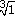• (b)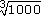• (a)= 1 since 13 = 1.

• (b)= 10 since 103 = 1000.

## Activity 12

Find the volume of a one-foot cube in cubic metres (1 foot = 30.48 cm). Estimate your answer first. Round your answer to three decimal places.

To estimate an answer, choose a simple approximation, say

1 foot30 cm = 0.3 m.

Then 1 foot cubed(0.3)3 = 0.027 m3.

More accurately, 1 foot = 30.48 cm = 0.3048 m.

So 1 foot cubed = (0.3048)3 = 0.028 m3 to three d.p.

## Activity 13

Without using your calculator, find the following, as estimates for the calculator work in Question 7.

• (a) 92

• (b) 43

• (c)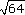• (d)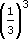• (e) (3)2

• (a) 92 = 9 × 9 = 81

• (b) 43 = 4 × 4 × 4 = 16 × 4 = 64

• (c)= 8 (since 82 = 64)

• (d)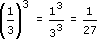• (e) (3)2 = 3 × 3 = 9

## Activity 14

• (a) 9.422

• (b) 3.653

• (c)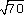• (d) 0.333

• (e) (2.713)2

• (b) 3.65348.6271
• (c)8.3666
• (d) 0.3330.0359 (check this by calculating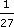, the estimate from 3(d) above, as a decimal.
• (e) (2.713)27.3604 (N.B. To get this answer on a calculator, remember the brackets.)